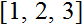# Volume 13, 2007, Number 1

Volume 13 ▶ Number 1 ▷ Number 2Number 3Number 4

A note on rhotrix exponent rule and its applications to some special series and polynomial equations defined over rhotrices
Original research paper. Pages 1—15
A. Mohammed
Full paper (138 Kb) | Abstract

This paper establishes and characterizes the theorem on rhotrix exponent rule, and presents the theory to stimulate systematization of expressing some special series and polynomial equations in terms of the relatively new method of representing arrays of real numbers.

Odd powers as sums of squares
Original research paper. Pages 16—24
J. V. Leyendekkers and A. G. Shannon
Full paper (113 Kb) | Abstract

Odd integers raised to an odd power n can equal a sum of squares only if the integers are in Class ̅14 of the Modular Ring Z4. The primes raised to an odd power are unique in that they only have (n − 1) square couples. These couples depend on the single couple (a1, b1). A general equation is given for predicting the square couples of pn and odd-powered composites. Even integers raised to an odd power have no primitive solutions for such square couples, because the sum of two odd squares falls in Class ̅24 where there are no powers at all.

Some generalized rising binomial coefficients
Original research paper. Pages 25—30
A. G. Shannon
Full paper (95 Kb) | Abstract

This paper considers some properties of generalized binomial coefficients which are defined in terms of rising factorial coefficients. Analogies with classical results in number theory and some generalized special functions are highlighted.

On some Pascal’s like triangles. Part 1
Original research paper. Pages 31—36
Krassimir T. Atanassov
Full paper (103 Kb) | Abstract

In a series of papers we shall discuss new types of Pascal’s like triangles. Triangles from the present form, but not with the present sense, are described in different publications, e.g., but at least the author had not found a research with similar idea. In the first part of our research we shall study properties of \standard” sequences, while in the next parts the objects of our interest will be more nonstandard sequences.

Volume 13 ▶ Number 1 ▷ Number 2Number 3Number 4